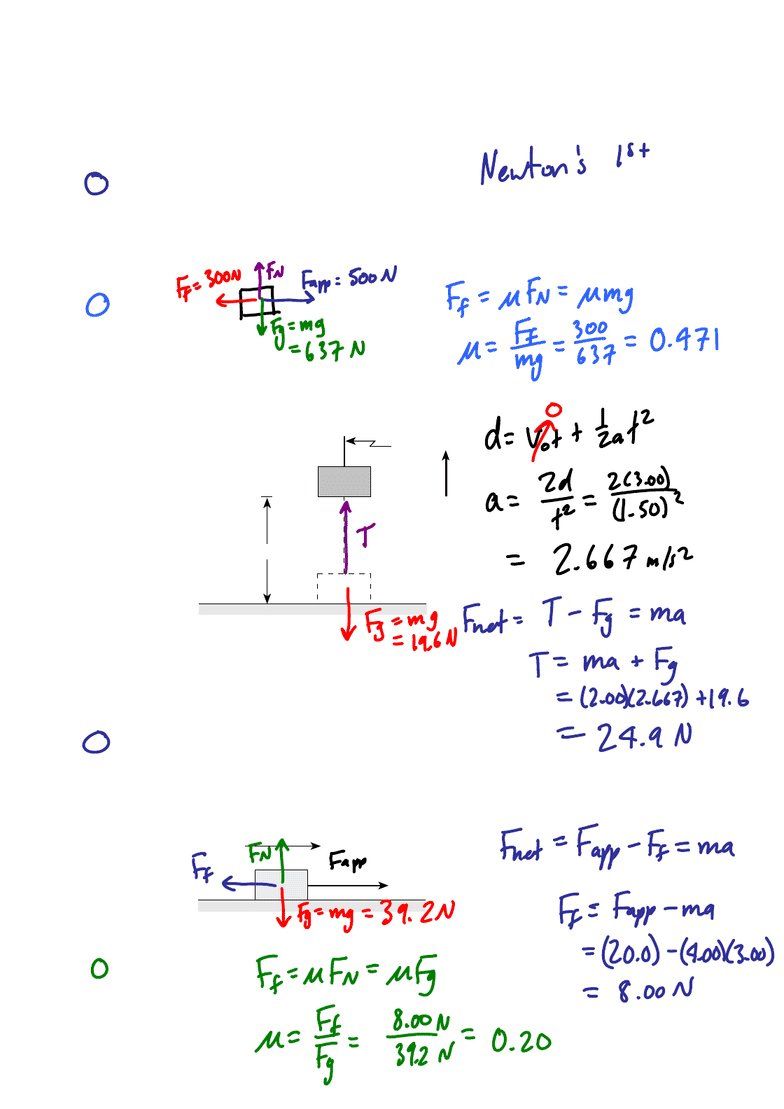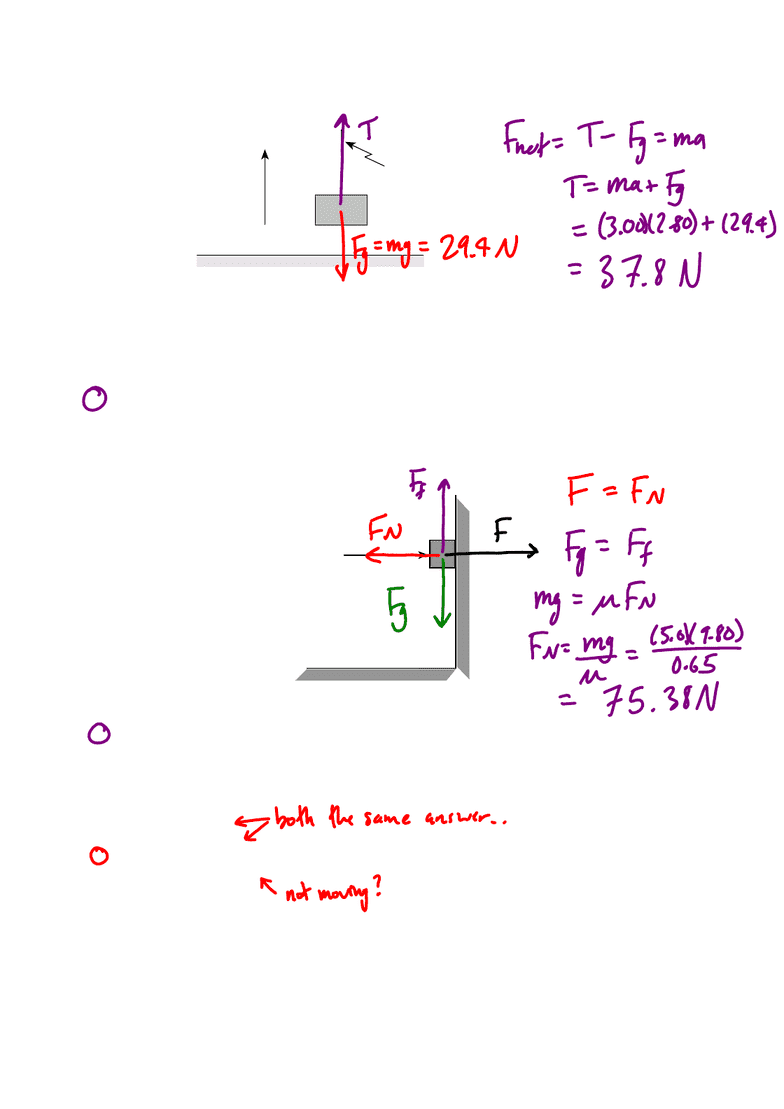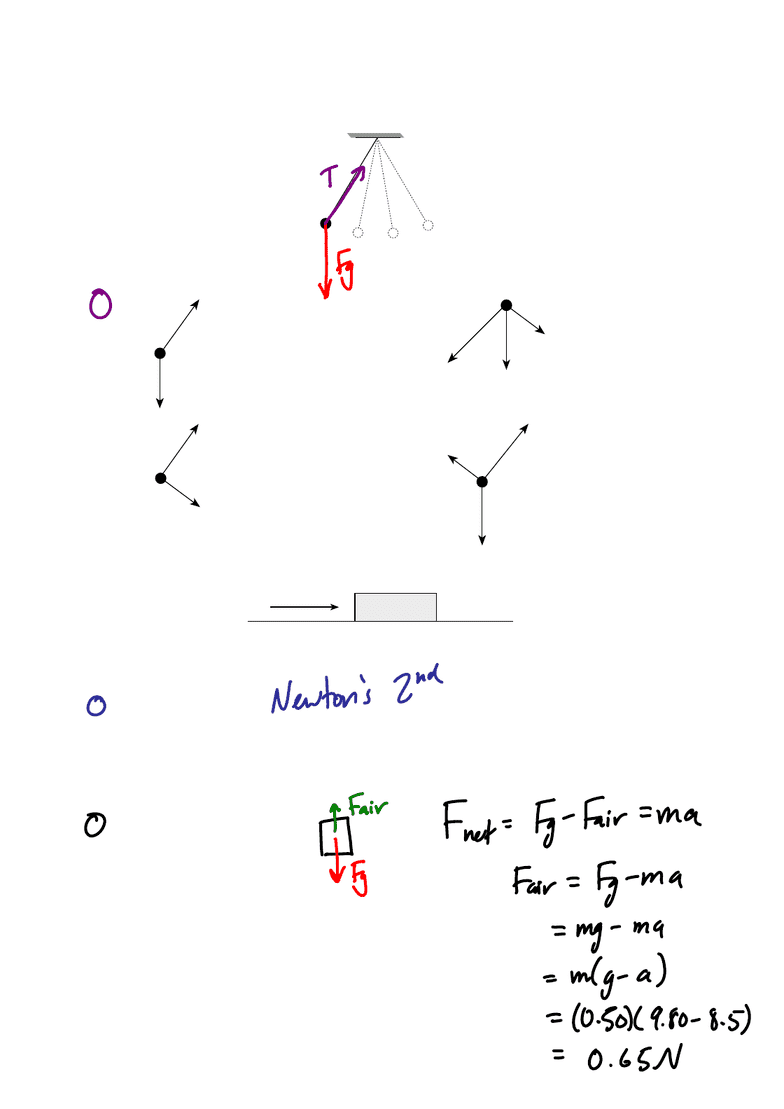# PHYS 221 Lecture Notes - Lecture 1: Sin 34, Mississippi Highway 15, Free Body Diagram

300 views16 pages- 1 -
1. Unless acted on by an external net force, an object will stay at rest or
A. come to rest.
B. decelerate at a constant rate.
C. slow down from a given speed.
D. continue to move in a straight line at a constant speed.
2. A 65.0 kg block is being accelerated along a level surface. The applied force is 500 N and the
friction force is 300 N. What is the coefficient of friction between the block and the surface?
A. 0.31
B. 0.47
C. 0.78
D. 1.30
3. A 2.00 kg object, initially at rest on the ground, is accelerated vertically by a rope, as shown.
The object reaches a height of 3.00 m in 1.50 s.
2.00 kg
rope
ground
3.00 m
What is the tension in the rope during the acceleration?
A. 5.33 N
B. 14.3 N
C. 23.6 N
D. 24.9 N
4. A 4.00 kg block is accelerated along a level surface at 3.00 m/s
2
. The applied force is 20.0 N.
3.00 m/s
2
20.0 N
4.00 kg
What is the coefficient of friction between the block and the surface?
A. 0.20
B. 0.31
C. 0.51
D. 0.67
Unlock document

This preview shows pages 1-3 of the document.
Unlock all 16 pages and 3 million more documents.5. A 3.00 kg object is being accelerated vertically upwards at 2.80 m/s
2
, as shown.
3.00 kg
cord
ground
2.80 m/s
2
What is the tension in the cord?
A. 8.40 N
B. 21.0 N
C. 29.4 N
D. 37.8 N
6. What minimum horizontal force F will just prevent the 5.0 kg block from sliding if the coefficient
of friction between the wall and the block is 0.65?
F
A. 6.4 N
B. 32 N
C. 49 N
D. 75 N
7. An object is sliding down a smooth incline. If friction is negligible, the object has
A. constant velocity.
B. constant momentum.
C. constant acceleration.
D. constant displacement.
- 2 -
Unlock document

This preview shows pages 1-3 of the document.
Unlock all 16 pages and 3 million more documents.8. A pendulum is swinging freely between points R and S as shown in the diagram below.
RS
Which of the following diagrams best represents the forces acting on the pendulum bob at point R?
A. B.
C. D.
9. A constant force is applied to an object on a frictionless surface, as shown in the diagram below.
Force
The resulting motion has
A. constant velocity.
B. constant momentum.
C. constant acceleration.
D. constant kinetic energy.
10. What is the frictional force due to air resistance on a 0.50 kg object falling vertically with an
acceleration of 8.5 m/s
2
?
A. 0.65 N
B. 4.3 N
C. 4.9 N
D. 9.2 N
- 3 -
Unlock document

This preview shows pages 1-3 of the document.
Unlock all 16 pages and 3 million more documents.